Construction of a circle when its radius is known

Chapter 14 Class 6 Practical Geometry
Concept wise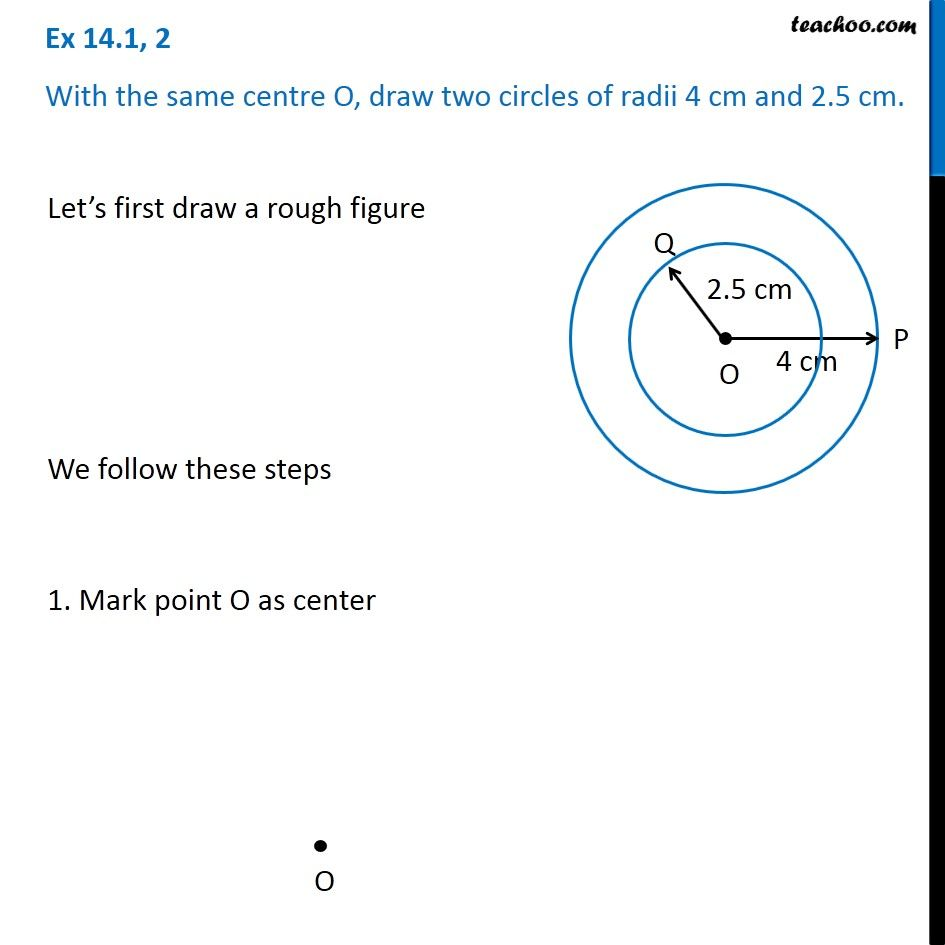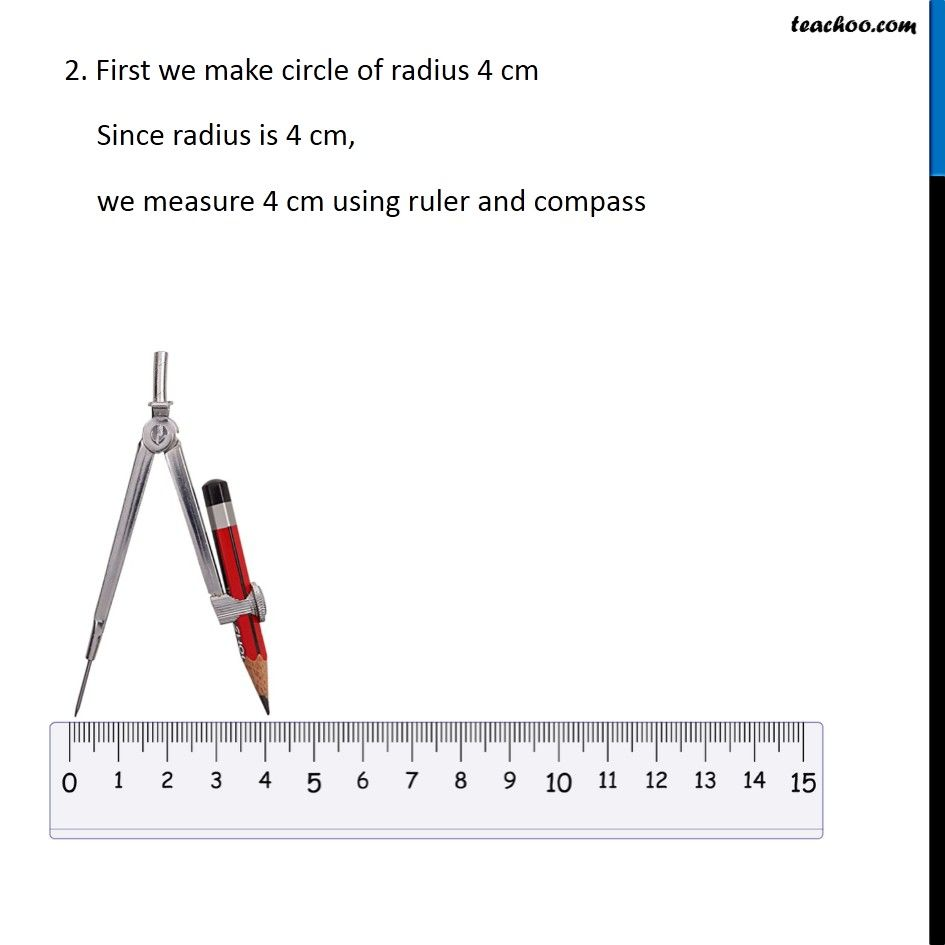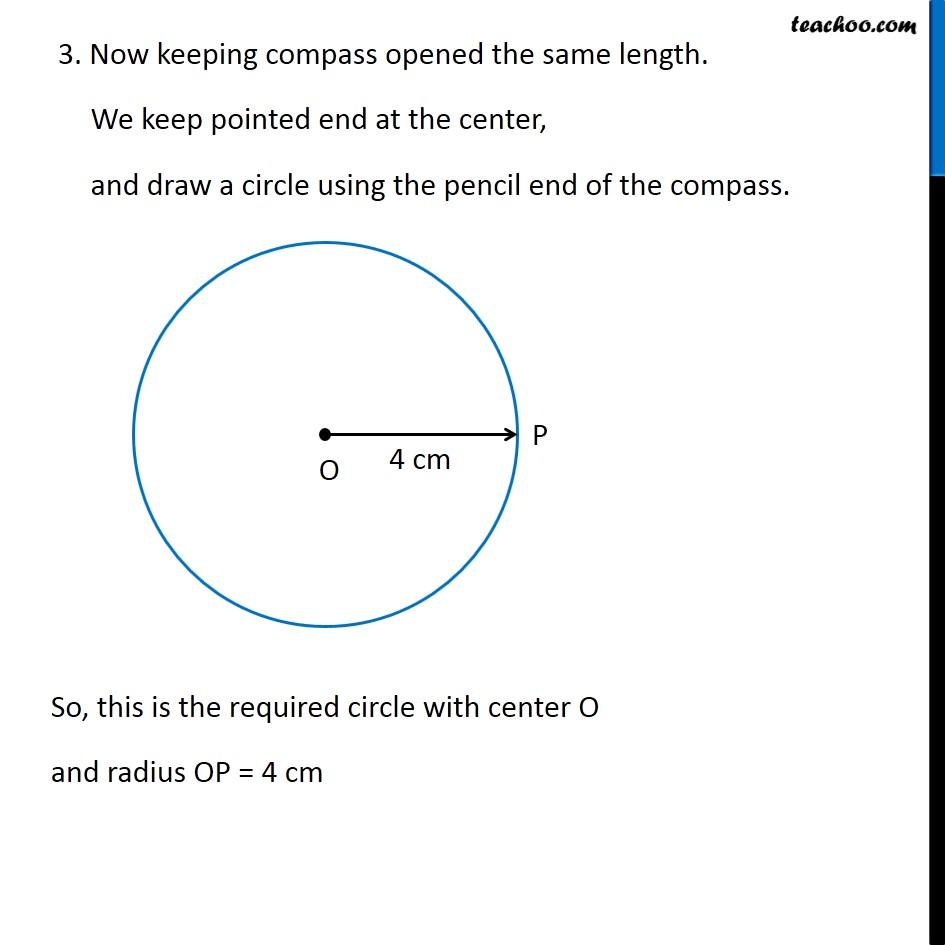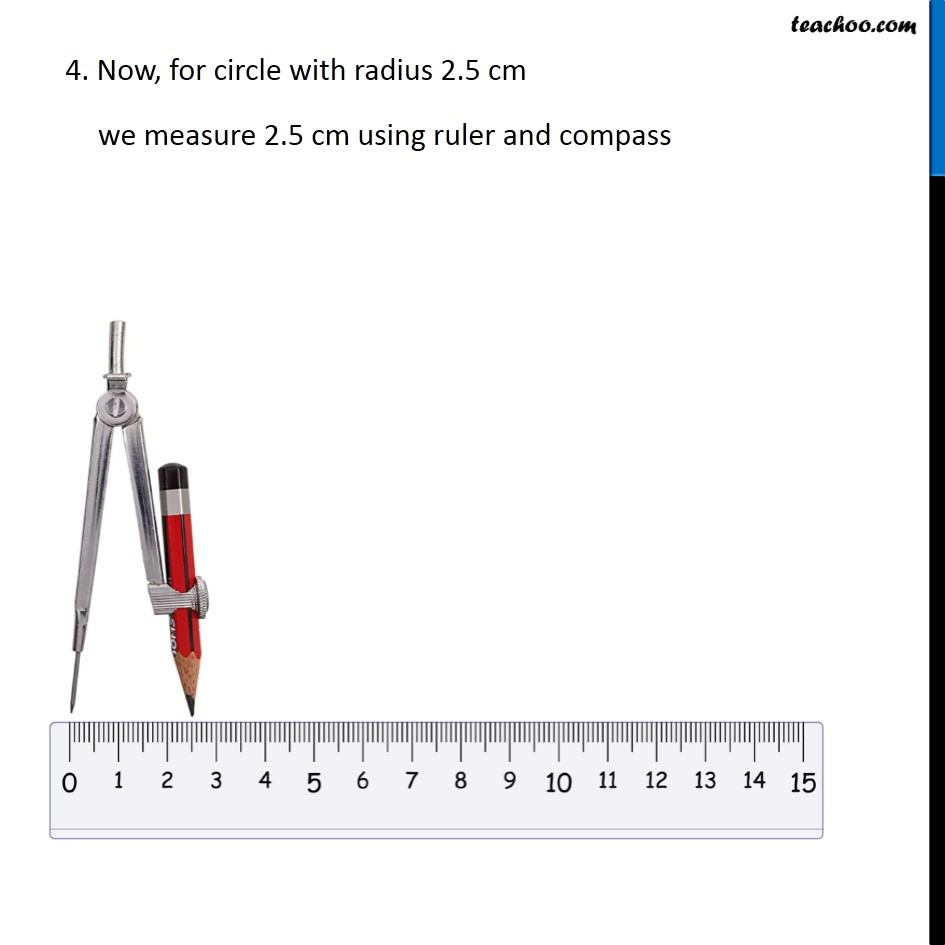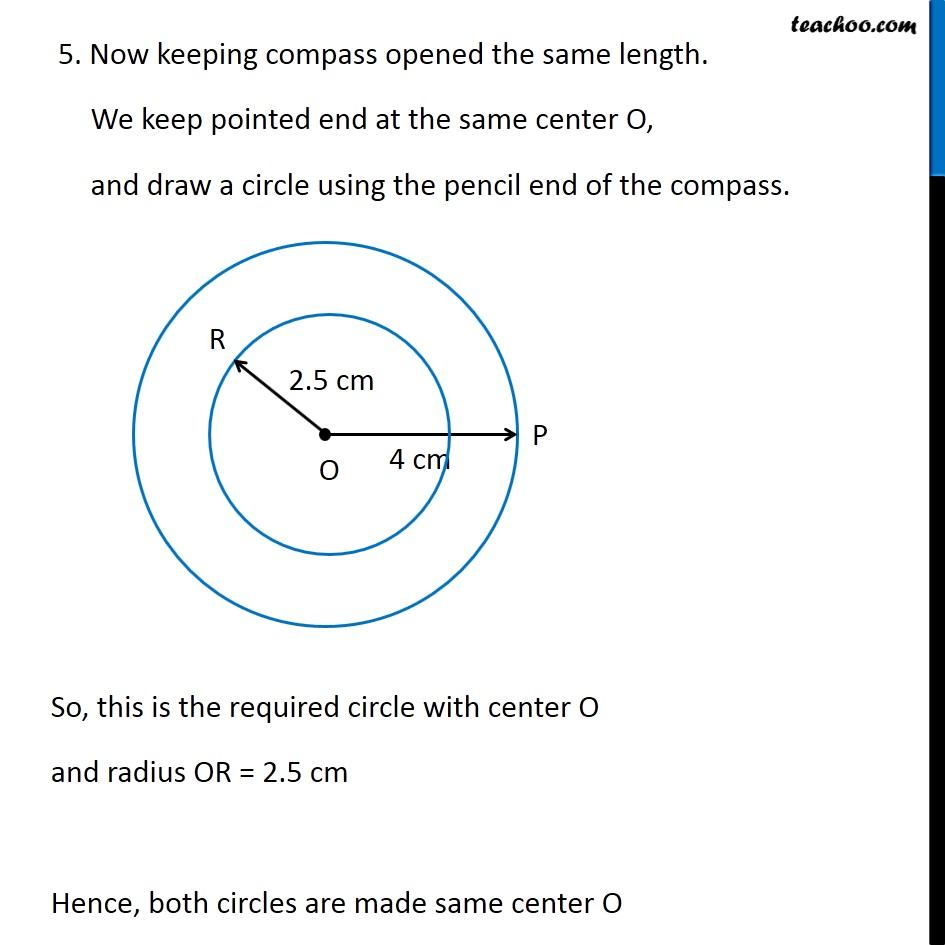### Transcript

Ex 14.1, 2 With the same centre O, draw two circles of radii 4 cm and 2.5 cm. Let’s first draw a rough figure We follow these steps 1. Mark point O as center 2. First we make circle of radius 4 cm Since radius is 4 cm, we measure 4 cm using ruler and compass 3. Now keeping compass opened the same length. We keep pointed end at the center, and draw a circle using the pencil end of the compass. So, this is the required circle with center O and radius OP = 4 cm 4. Now, for circle with radius 2.5 cm we measure 2.5 cm using ruler and compass 5. Now keeping compass opened the same length. We keep pointed end at the same center O, and draw a circle using the pencil end of the compass. So, this is the required circle with center O and radius OR = 2.5 cm Hence, both circles are made same center O